• fft2
千次阅读
2021-04-26 17:01:01

计算二维离散傅立叶变换

此函数通过快速傅立叶变换(FFT)计算M-dimensional阵列中任何轴上的n-dimensional离散傅立叶变换。默认情况下，该变换是在输入数组的最后两个轴上进行计算的，即二维FFT。

参数：

x：array_like输入数组，可能很复杂

s：sequence of ints, 可选参数输出的形状(每个变换轴的长度)s指轴0，s到轴1等)。这对应于n对于fft(x, n)。沿每个轴，如果给定的形状小于输入的形状，则将裁剪输入。如果较大，则输入将填充零。如果未指定s，则使用沿轴指定的轴的输入形状。

axes：sequence of ints, 可选参数计算FFT的轴。如果未给出，则使用最后两个轴。

norm：{None, “ortho”}, 可选参数规范化模式(请参阅fft)。默认为无。

overwrite_x：bool, 可选参数如果为True，则x的内容可以被销毁；默认值为False。参考fft更多细节。

workers：int, 可选参数用于并行计算的最大工人数。如果为负，则值从os.cpu_count()。参考fft更多细节。

返回值：

异常：

ValueError如果s和轴的长度不同，或者未指定轴和len(s) != 2。

IndexError如果轴的元素大于x的轴数。

注意：

fft2只是fftn轴的默认值不同。

输出类似于fft，在转换轴的低阶角包含零频率项，在这些轴的前半部分包含正频率项，在轴中间包含奈奎斯特频率项，在第二个轴包含负频率项轴的一半，以负频率递减的顺序排列。

参考fftn有关详细信息和绘图示例，以及fft用于使用的定义和约定。

例子：

>>> import scipy.fft

>>> x = np.mgrid[:5, :5]

>>> scipy.fft.fft2(x)

array([[ 50. +0.j , 0. +0.j , 0. +0.j , # may vary

0. +0.j , 0. +0.j ],

[-12.5+17.20477401j, 0. +0.j , 0. +0.j ,

0. +0.j , 0. +0.j ],

[-12.5 +4.0614962j , 0. +0.j , 0. +0.j ,

0. +0.j , 0. +0.j ],

[-12.5 -4.0614962j , 0. +0.j , 0. +0.j ,

0. +0.j , 0. +0.j ],

[-12.5-17.20477401j, 0. +0.j , 0. +0.j ,

0. +0.j , 0. +0.j ]])

更多相关内容
• FFT2 优化。 许多图像处理应用程序需要大量使用具有相同维度的矩阵的 FFT2 例程（或者，在最一般的情况下，N 维 FFT）。 在这些情况下，MATLAB FFT2 会导致效率极低。 通常，通过将 N 维 FFT 拆分为多个一维 FFT，...matlab
• 该笔记详细解释了如何应用 Matlab 二维 FFT 来过滤二维信号，例如图像。 展示了如何将 fft2 的输出连接到信号的实际傅立叶变换，特别是如何对简单滤波器的传递函数进行编码。matlab
• fft2函数源码数字图像处理简介。 关于 该项目显示了我认为对于希望了解数字图像处理的任何人都必须的想法。 要求： 结石 线性代数 可能性 具有图像处理包的Matlab或Octave 作业1-直方图形状。 在这些分配中，我们...
• matlab中的二维离散傅里叶变换函数fft2.m源码
• FFT spectrum analiz matlab script
• how to use fft2介绍了使用fft2要注意的问题
• 图像的二维傅里叶变换，matlab编程，很好用。
• 实现定点化8点FFT计算,函数内部用fi()构造定点数对象。
• ## fft2

千次阅读 2020-01-05 11:13:12
fft2 :二维快速傅里叶变换 语法 Y = fft2(X) Y = fft2(X,m,n) 说明 Y = fft2(X) 使用快速傅里叶变换算法返回矩阵的二维傅里叶变换，这等同于计算 fft(fft(X).’).’。如果 X 是一个多维数组，fft2 将采用高于 2 的...

### 文章目录

fft2 :二维快速傅里叶变换

## 语法

Y = fft2(X)
Y = fft2(X,m,n)


## 说明

Y = fft2(X) 使用快速傅里叶变换算法返回矩阵的二维傅里叶变换，这等同于计算 fft(fft(X).’).’。如果 X 是一个多维数组，fft2 将采用高于 2 的每个维度的二维变换。输出 Y 的大小与 X 相同。

Y = fft2(X,m,n) 将截断 X 或用尾随零填充 X，以便在计算变换之前形成 m×n 矩阵。Y 是 m×n 矩阵。如果 X 是一个多维数组，fft2 将根据 m 和 n 决定 X 的前两个维度的形状。

## 示例

二维傅里叶变换对处理二维信号和其他二维数据（如图像）很有用。

创建并绘制具有重复块的二维数据。

P = peaks(20);
X = repmat(P,[5 10]);
imagesc(X)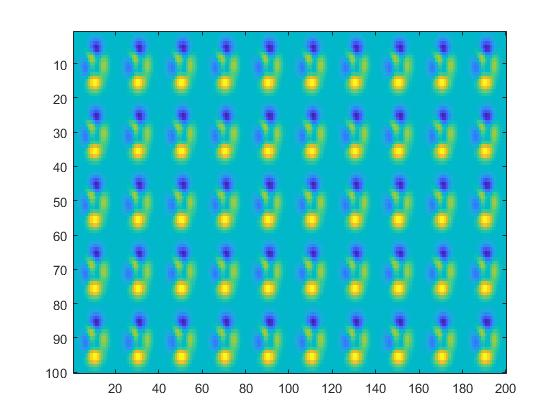计算数据的二维傅里叶变换。将零频分量移动到输出的中心，并绘制生成的 100×200 矩阵，它与 X 的大小相同。

Y = fft2(X);
imagesc(abs(fftshift(Y)))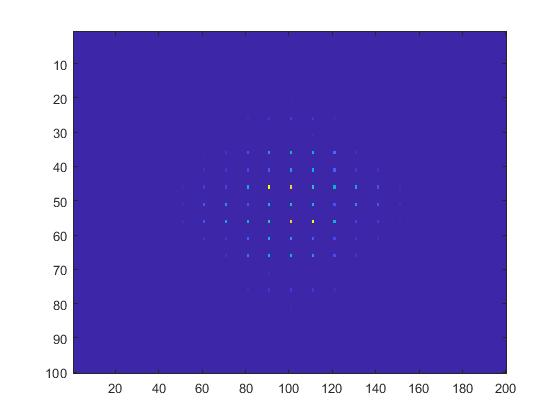用零填充 X 以计算 128×256 变换。

Y = fft2(X,2^nextpow2(100),2^nextpow2(200));
imagesc(abs(fftshift(Y)));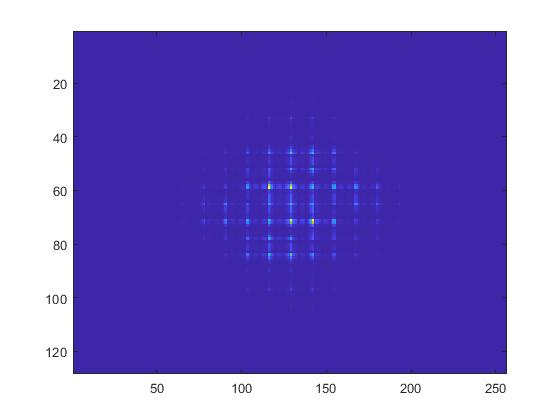展开全文• 该程序实现二维的FFT变换，可以对图像做FFT变换，运行速度还可以。
• 用C语言实现二维傅里叶变换，以及余弦变换，个人觉得好用。
• FFT应用举例 x=0.5*sin(2*pi*15*t)+2*sin(2*pi*40*t),fs=100Hz,绘制： （1）数据个数N=32，FFT所用的采样点数NFFT=32； （2）N=32，NFFT=128； （3）N=136，NFFT=128； （4）N=136，NFFT=512。
• Matlab 函数 fft、fft2 和 fftn 分别可以实现一维、二维和 N 维 DFT 算法；而函数 ifft、ifft2 和 ifftn 则用来计算反 DFT
• python的numpy库中的图像傅里叶变换公式： #计算一维傅里叶变换 numpy.fft.fft(a, n=None, axis=-1, norm=None) #计算二维的傅里叶变换 numpy.fft.fft2(a, n=None, axis=-1, norm=None) #计算n维的傅里叶变换 numpy...

码字不易，如果对您有所帮助，记着点赞哦！

一. 图像傅里叶变换原理：

对二维图像进行傅里叶变换用如下公式进行：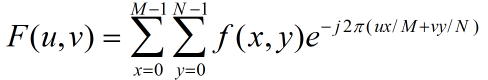图像长M，高N。F(u,v)表示频域图像，f(x,y)表示时域图像。u的范围为[0,M-1]，v的范围为[0,N-1]  ↑

对二维图像进行傅里叶逆变换用如下公式进行：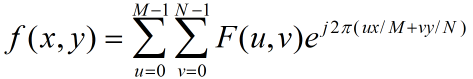图像长M，高N。f(x,y)表示时域图像， F(u,v)表示频域图像。x的范围为[0,M-1]，y的范围为[0,N-1]  ↑

二. python的numpy库中的图像傅里叶变换公式：

#计算一维傅里叶变换

numpy.fft.fft(a, n=None, axis=-1, norm=None)

#计算二维的傅里叶变换

numpy.fft.fft2(a, n=None, axis=-1, norm=None)

#计算n维的傅里叶变换

numpy.fft.fftn()

#计算n维实数的傅里叶变换

numpy.fft.rfftn()

#返回傅里叶变换的采样频率

numpy.fft.fftfreq()

#将FFT输出中的直流分量移动到频谱中央

numpy.fft.shift()

三. 实验：python的numpy库实现图像傅里叶变换及反变换

# writer:wojianxinygcl@163.com

# date  : 2020.3.30

import cv2 as cv

import numpy as np

from matplotlib import pyplot as plt

#读取图像

#傅里叶变换

f = np.fft.fft2(img)

fshift = np.fft.fftshift(f)

res = np.log(np.abs(fshift))

#傅里叶逆变换

ishift = np.fft.ifftshift(fshift)

iimg = np.fft.ifft2(ishift)

iimg = np.abs(iimg)

#展示结果

plt.subplot(131), plt.imshow(img, 'gray'), plt.title('Original Image')

plt.axis('off')

plt.subplot(132), plt.imshow(res, 'gray'), plt.title('Fourier Image')

plt.axis('off')

plt.subplot(133), plt.imshow(iimg, 'gray'), plt.title('Inverse Fourier Image')

plt.axis('off')

plt.show()

四. 实验结果：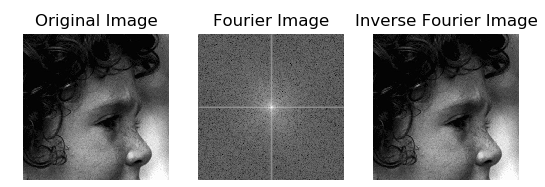第三部分代码输出结果 ↑

五. python的cv2库中的图像傅里叶变换公式：

# 傅里叶变换

dft = cv2.dft(np.float32(img), flags = cv2.DFT_COMPLEX_OUTPUT)

# 傅里叶逆变换

iimg = cv2.idft(ishift)

# 返回傅里叶变换后iimg的幅值

res2 = cv2.magnitude(iimg[:,:,0], iimg[:,:,1])

六. 实验：python的cv库和numpy库实现图像傅里叶变换及反变换

# writer:wojianxinygcl@163.com

# date  : 2020.3.30

import numpy as np

import cv2

from matplotlib import pyplot as plt

#读取图像

#傅里叶变换

dft = cv2.dft(np.float32(img), flags = cv2.DFT_COMPLEX_OUTPUT)

dftshift = np.fft.fftshift(dft)

res1= 20*np.log(cv2.magnitude(dftshift[:,:,0], dftshift[:,:,1]))

#傅里叶逆变换

ishift = np.fft.ifftshift(dftshift)

iimg = cv2.idft(ishift)

res2 = cv2.magnitude(iimg[:,:,0], iimg[:,:,1])

#显示图像

plt.subplot(131), plt.imshow(img, 'gray'), plt.title('Original Image')

plt.axis('off')

plt.subplot(132), plt.imshow(res1, 'gray'), plt.title('Fourier Image')

plt.axis('off')

plt.subplot(133), plt.imshow(res2, 'gray'), plt.title('Inverse Fourier Image')

plt.axis('off')

plt.show()

七. 实验结果：第六部分代码输出结果 ↑

八. 参考内容：

九. 版权声明：

未经作者允许，请勿随意转载抄袭，抄袭情节严重者，作者将考虑追究其法律责任，创作不易，感谢您的理解和配合！

展开全文python源码 数字图像处理
• c语言 2D-FFT(fft2)及IFFT.pdf
• 基于TMS320C6455的二维FFT实现 - dsplib_c64Px_3_4_0_0_Win32.exe ... - FFT2 - FFT2实现工程 - TI Compiler v7.4.18 - ImageResize - 用来调整图像尺寸并生成对应code的Python脚本 - 依赖opencv-python
• torch.fft.fft2.() 报错问题解决 问了别人，应该是fft函数对应的torch版本问题，torch1.8.0版本之后的才是 torch.fft.fft2 根据网上的总结自己改的 #旧版 新版 torch.rfft torch.fft.fft2 torch.irfft torch...

运行别人的的源代码还报错，所以确定不是代码问题。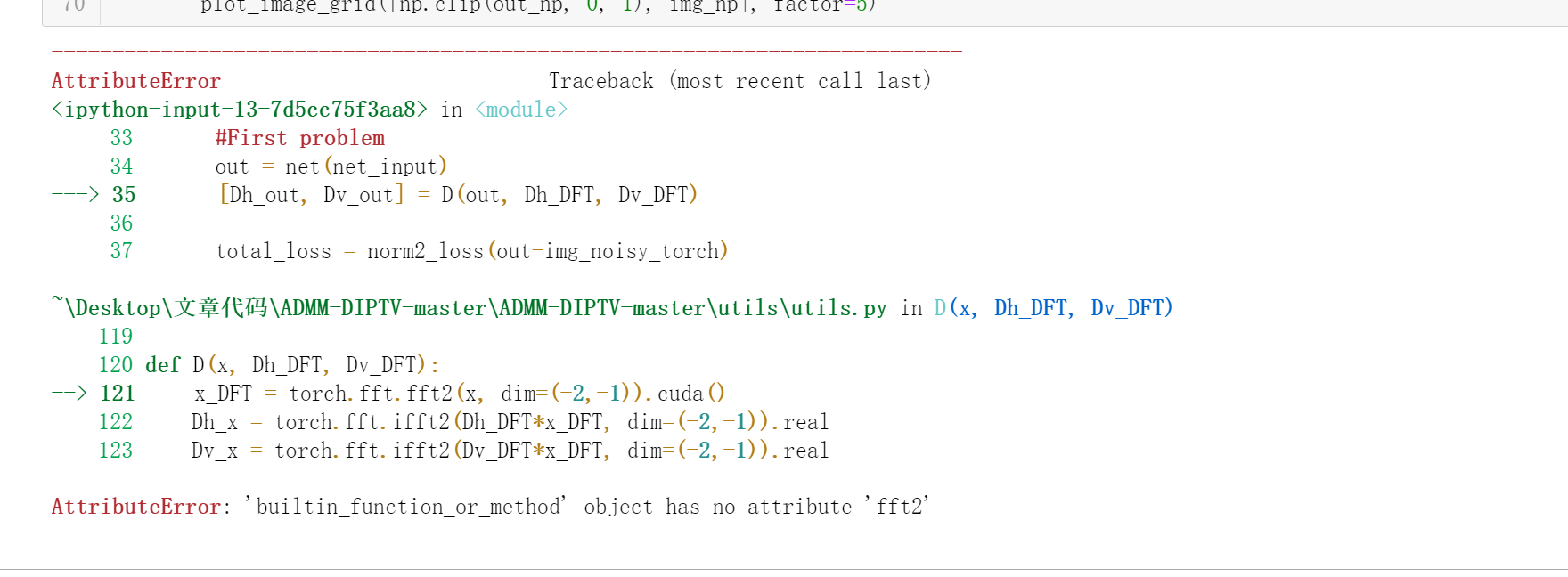# 问了别人，应该是fft函数对应的torch版本问题，torch1.8.0版本之后的才是

torch.fft.fft2

根据网上的总结自己改的

#旧版          新版
torch.rfft   torch.fft.fft2
torch.irfft  torch.fft.ifft2

还是报错了，函数中使用的参数定义应该也是不一样的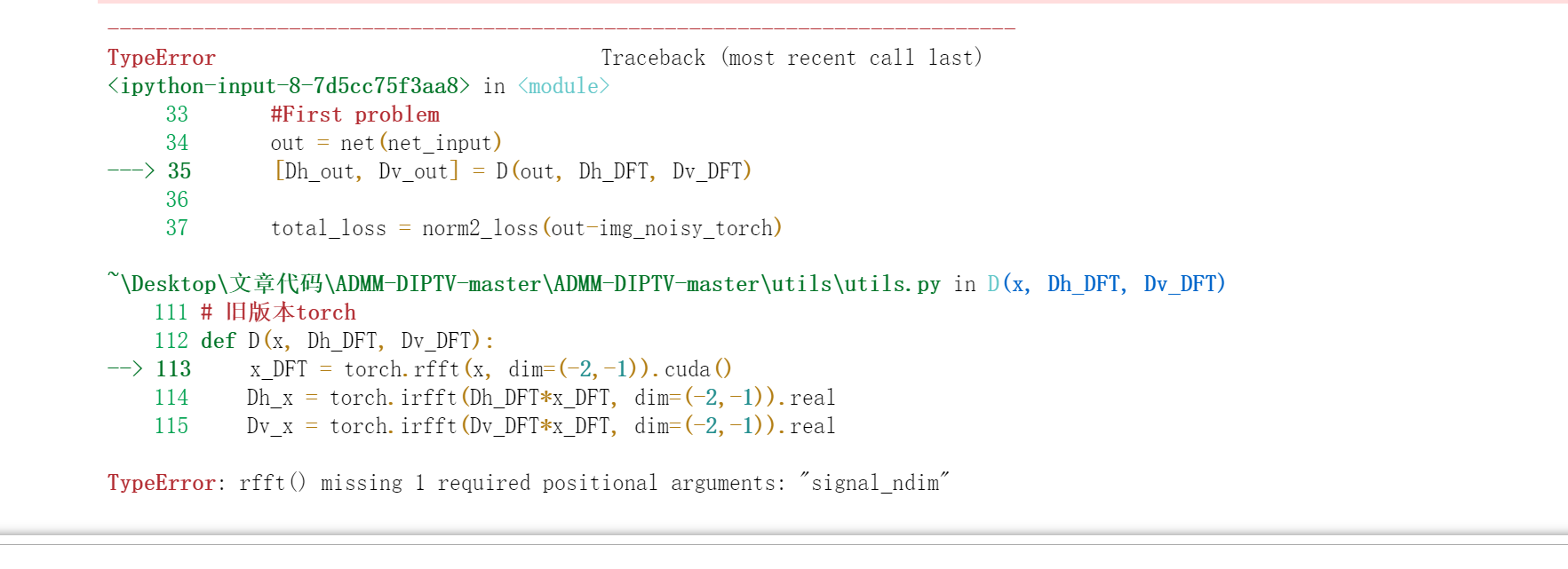原来不是版本问题，看到pytorch官网上只有这两种函数，所以猜想是不是我写错了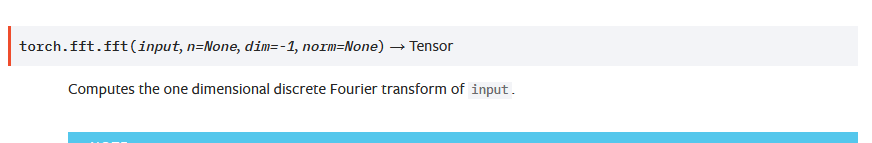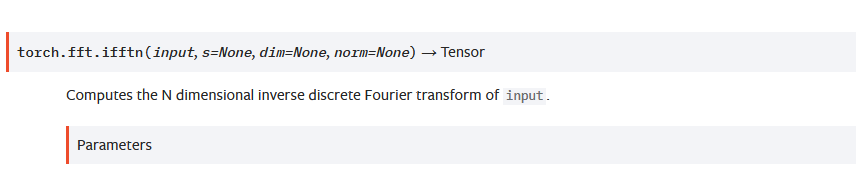# 解决办法，把源代码的torch.fft.fft2改成torch.fft.fftn就可以了

def D(x, Dh_DFT, Dv_DFT):
x_DFT = torch.fft.fftn(x, dim=(-2,-1)).cuda()
Dh_x = torch.fft.ifftn(Dh_DFT*x_DFT, dim=(-2,-1)).real
Dv_x = torch.fft.ifftn(Dv_DFT*x_DFT, dim=(-2,-1)).real
return Dh_x, Dv_x

然后代码正常运行啦，为了证明代码真的运行成功过，因为最近不知道怎么回事，又开始报和原来一样的错误了，找不到原因，但是至少成功过，那我的改的应该是对的。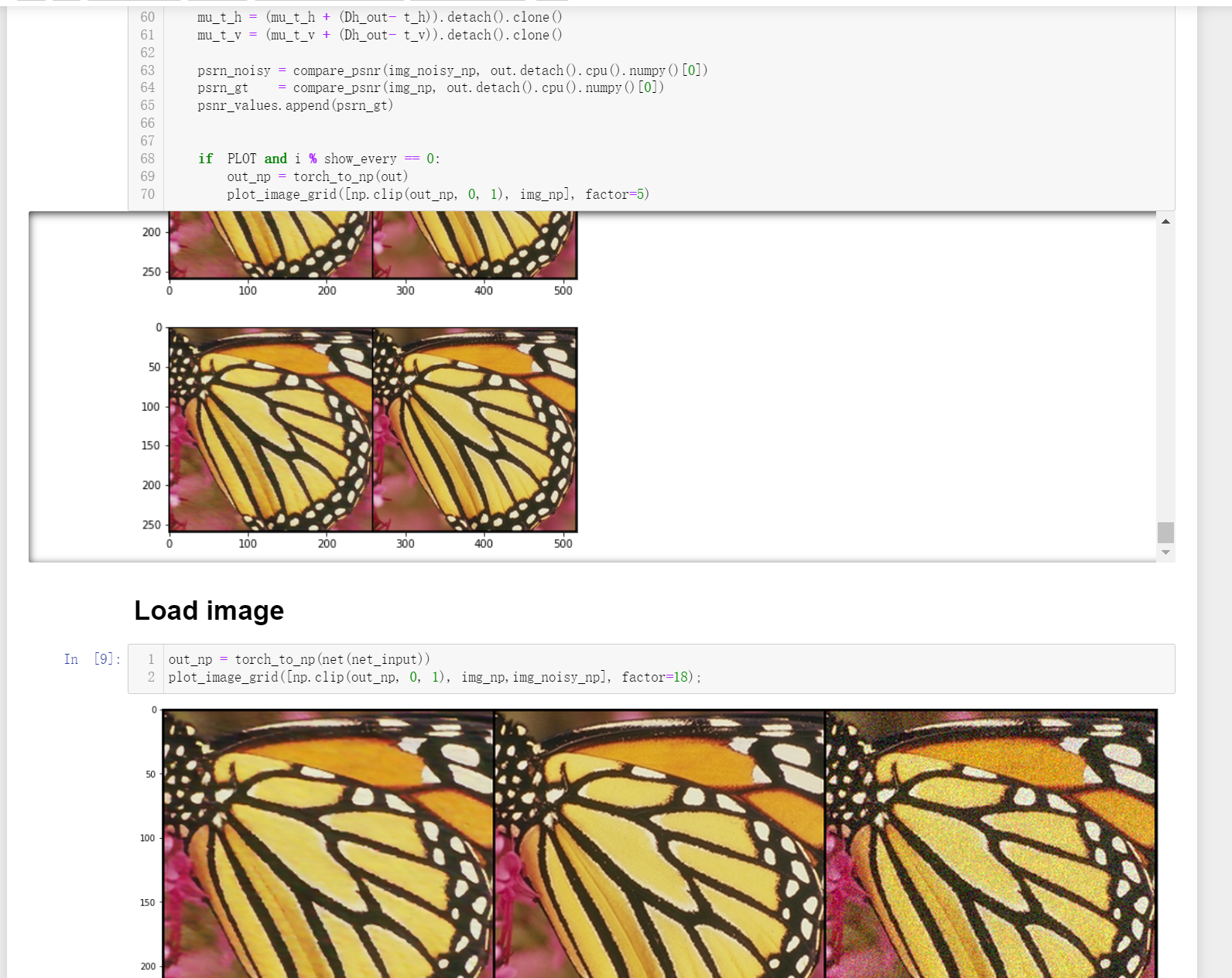展开全文python pytorch 深度学习
• 文章目录一、离散傅立叶变换二、离散余弦变换1.dct2 函数2.dict2 函数3.dctmtx 函数三、图像小波变换1.一维小波变换dwt函数idwt函数2.二维小波变换wcodemat函数dwt2函数wavedec2函数idwt2函数waverec2函数 一、离散...matlab
• 可是在图像处理方面，须要做二维变换，这个时候就须要用到FFT2.在利用Octave(或者matlab)里面的fft2()函数的时候，观察频率领域的图像还是要点额外的技巧的.以下的图像是我们想要的，也是我们人类才干够理解的(图片...
• 基于vHdl 的 fft变换的实现代码，可以参考
• 采用c语言编写的二维傅里叶变换，对想要采用硬件Fpga实现的同学有一定的参考价值，目前在xilinx开发板上实现sdk处理fft2，效果还不错
• 快速傅里叶变换 fft2 (Matlab) -> np.fft.fft2 数组滚动 circshift(Matlab) -> np.roll 事实上，对于三维数组，Matlab会将其分解其多个二维数组进行处理，而Python并不会这样（主要是针对图像处理过程中可能...
• 1.语法：         Y = fft2(X)         Y = fft2(X,m,n) 2.说明：    ...Y = fft2(X) 使用快速...matlab
• ## OpenCV实现Matlab的fft2、ifft2函数

千次阅读 多人点赞 2017-10-05 23:24:28
看标题就知道跟我的上一篇博客差不多了，本来没打算写这篇的，因为之前在网上搜过，有现成的OpenCV实现fft2和ifft2代码，而且我也试过fft2确实和Matlab计算的结果一致。但是项目后来又用到了ifft2，这次发现计算结果...matlab opencv
• 需要注意的是，在进行傅里叶变换（fft2）后，低频部分集中分布在四个角落，通过移位（fftshift）之后，将其集中到图像的中心，这只是为了便于处理。 当我们对幅值进行处理后，再进行反移位（ifftshift）恢复到原始...matlab 傅里叶变换 频率域去噪
• 如图 使用np.fft.fft2对图片进行傅里叶变换时，遇到了这样的错误。 请一定要查看图片路径是否正确，我就是因为图片路径没设置对才出现了这个错误。下面是错误代码，我误认为图片和程序在同一路径下其实并不是 img...python
• # -*- coding: utf-8 -*- """ Created on Sun Jul 8 21:05:51 2018 @author: Diko "...def FFT_v1(Img,Wr): if Img.shape==2: pic = numpy.zeros(,dtype=complex) pic...二维快速傅里叶变换 傅里叶变换...